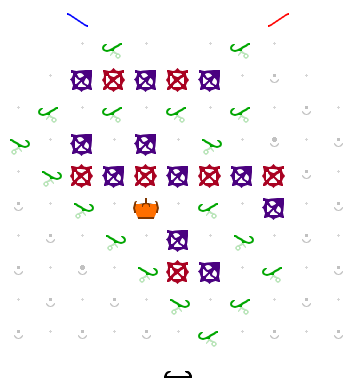# [Hard] Binary input - Divisible by four

Here’s another “binary input” challenge.

The challenge is to determine if a binary input is divisible by four. The binary input is entered by feeding one ball at the time, where each ball represents a bit, its value determined by its color. You can decide which color represent the 0-bit, and which represent the 1-bit. It does not really matter. The least significant bit is entered first. There is no restriction on the size of the input. At any time, the board should indicate if the number entered so far is divisible by four.

No prize for originality as the problem statement is very similar to the divisible-by-three problem posed by @lennypitt. However, establishing that there actually exists a solution on a normal-sized Turing Tumble board is not easy.

At first glance, the problem may not look that hard, given that four is a power of two. However, as you start solving it, I think you’ll find that it is not so straightforward. It requires a different approach than the divisible-by-three problem. The real difficulty is to devise a solution that fits on a normal-sized board. It is possible though. Can you manage?

I don’t have a solution, but I have a scheme that could lead to a solution:

insight: you don’t care about 0s after the first two, nor 1s after the first, so you’re looking for a widget that sends the first 1 somewhere then latches, a widget that sends the second 0 somewhere then latches, and a widget with 2 inputs, which decides what to do with the first based on whether the second has fired yet.

I’m pretty sure this gets you there, but it’s too late at night for me to break out the board…

Well, except for null input, and inputs of 0, and 00, I think this works. It is just checking that the first two bits are 0s. So, the same as what @rmsgrey suggests. If the two blue bits are set to 1, then the number is divisible by four. (It is problematic that null and “0” indicate not divisible by four, but “00” indicates divisible by four.)

EDIT: I can’t seem to get the saved url to behave. For some reason, it shows a misplaced gear. In the solution above, put a gear where there is clearly one missing, connecting the gearbits on the top left.

I’d be interested in seeing a solution when the bits are entered most-significant-bit-first. Hmm. I suppose then you just keep track of the last two bits, so perhaps that is not hard?

Interesting. However, the fact that it does not correctly classify null input, 0 and 00 I consider an error. Fortunately, that is not too hard to remedy. The device can start by assuming the number is divisible, and update its state when this is not the case anymore, i.e. when either the first bit or second bit is a 1 change its state. So either let the blue balls clear the bits, or swap the bitness coloring (i.e. let the red balls be the 1s).

There are two further improvements you can make. First, instead of having two bits which you need to “and” yourself, you can have a single bit indicate divisibility.

Furthermore, you can add pass-through, i.e. let all balls be collected in the same interceptor or fall through (possibly via a switch you can manually toggle to select the next bit).

Below is my solution. Note, the red balls are the 1s, the blue balls the 0s.https://www.lodev.org/jstumble/?board=llbgbgbellllerbbreergbgbgbgeerxlbeerbrfrgblfrlfeleei

The bottom bit indicates if the number is divisible. It is initially set to “true” (as zero is divisible by four). Once set to “false”, this will not change. The middle bit also starts “true”, and can also be permanently set to “false”. It returns “true” as long as a 1-bit impacts divisibility. The top bit is used to set the next bit to “false” at the right moment.

Yes - 0, 00 are errors. I got tired(Not sure how to treat null, or whether “00” is a fair input, since we don’t write numbers with leading 0s. We don’t have this problem if we’re entering numbers most significant bit first.)

Now that I’m better rested, here’s a sample layout:

https://www.lodev.org/jstumble/?board=rregae0eabbgreexgbble1rrlererleerelfrlfeiz

The first blue ball (1) and second red ball (0) both get diverted to the middle gears. If the blue ball gets there first, then the signal bit (lower bit) gets flipped to “false”; if the red ball gets there first, then the blue ball passes through harmlessly. All other balls go straight into the collection paths.

2 Likes

That’s ingenious! I considered various variants on counting bits, but had not considered separately counting the 0s and 1s. That really simplifies things.

Btw, there’s still a minor mistake in your solution. The lowest bit should be a simple ramp.

The lowest bit is the output - true until a blue is input before 2 reds have been input (so *1 and *10 are the only strings that output false)

Ah, you’re right. I mistakenly thought that the bit that feeds it was tracking divisibility, but now see that it is still a routing gadget. The source of my confusion was the empty exit at the right of the bottom bit, but it’s now clear that no ball will ever exit from that side.